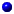M408M Learning Module Pages
Main page

### Chapter 12: Vectors and the Geometry of Space

#### Learning module LM 12.6: Surfaces:

Surfaces and traces
Level curves
Level surfaces
Worked problems

# Level curves

Level Curves
For a general function $z = f(x,\,y)$, slicing horizontally is a particularly important idea:

 Level curves: for a function $z=f (x,\,y) :\, D \subseteq {\mathbb R}^2 \to {\mathbb R}$ the level curve of value $c$ is the curve $C$ in $D \subseteq {\mathbb R}^2$ on which $f\Bigl|_{C} = c\,$.

Notice the critical difference between a level curve $C$ of value $c$ and the trace on the plane $z = c$: a level curve $C$ always lies in the $xy$-plane, and is the set $C$ of points in the $xy$-plane on which $f(x,\,y) = c$, whereas the trace lies in the plane $z = c$, and is the set of points $(x,\,y,\, c)$ with $(x,\,y)$ in $C$.

By combining the level curves $f(x,\,y) = c$ for equally spaced values of $c$ into one figure, say $c = -1, \,0,\, 1,\, 2,\, \ldots \,,$ in the $xy$-plane, we obtain a contour map of the graph of $z=f(x,\,y)$. Thus the graph of $z = f(x,\,y)$ can be visualized in two ways,one as a surface in $3$-space, the graph of $z = f(x,\,y)$,the other as a contour map in the $xy$-plane, the level curves of value $c$ for equally spaced values of $c$.
As we shall see, both capture the properties of $z = f(x,\,y)$ from different but illuminating points of view. The particular cases of a hyperbolic paraboloid and a paraboloid are shown interactively in

 Problem: Describe the contour map of a plane in $3$-space. Solution: The equation of a plane in $3$-space is $$Ax + By + Cz \ = \ D\,,$$ so the horizontal plane $z= c$ intersects the plane when $$Ax + By +Cc \ = \ D\,.$$ For each $c$, this is a line with slope $-A/B$ and $y$-intercept $y = (D-Cc)/B$. Since the slope does not depend on $c$, the level curves are parallel lines, and as $c$ runs over equally spaced values these lines will be a constant distance apart. Consequently, the contour map of a plane consists of equally spaced parallel lines. (Does this make good geometric sense?)